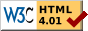## Dokumentation zu: set_bit(E)``` SYNOPSIS string set_bit(string str, int n) DESCRIPTION Return the new string where bit n is set in string str. Note that the old string str is not modified. Each character contains 6 bits. So you can store a value between 0 and 63 in one character (2^6=64). Starting character is the blank " " which has the value 0. The first charcter in the string is the one with the lowest bits (0-5). The new string will automatically be extended if needed. EXAMPLES string s; s=set_bit("?",5); Because "?" has a value of 31 the variable s will now contain the character "_" which is equal to 63 (31+2^5=63). string s; s=set_bit("78",3); s=set_bit(s,8); s will now contain the string "?<". SEE ALSO clear_bit(E), last_bit(E), next_bit(E), test_bit(E), count_bits(E), and_bits(E), or_bits(E), xor_bits(E), invert_bits(E), copy_bits(E) ``` Die Seite ist auch in Deutsch vorhanden.

Start » Magierhandbuch » Docu » Efun » Set_bit Letzte Generierung: 25.04.2021, 01:58mud@wl.mud.de